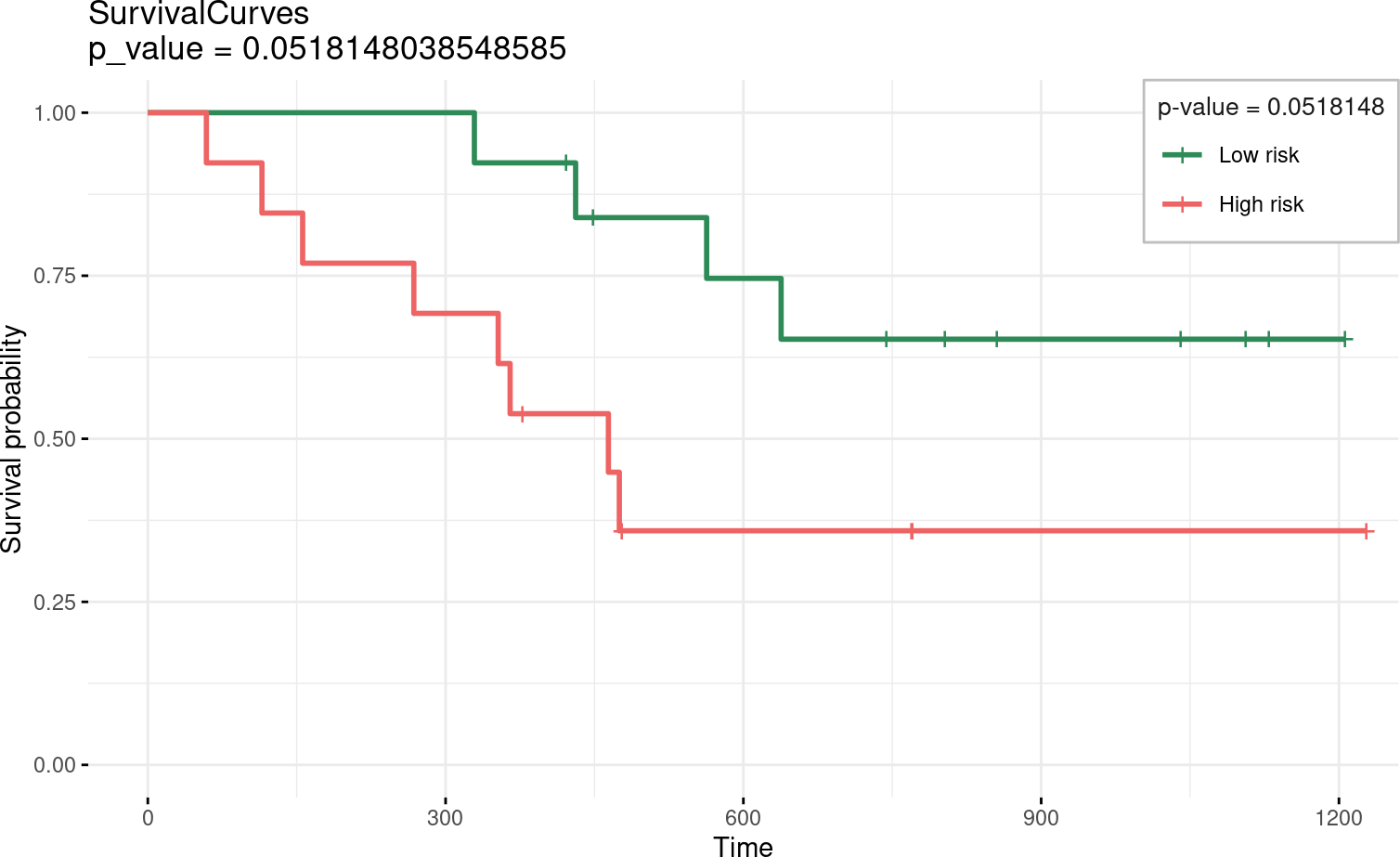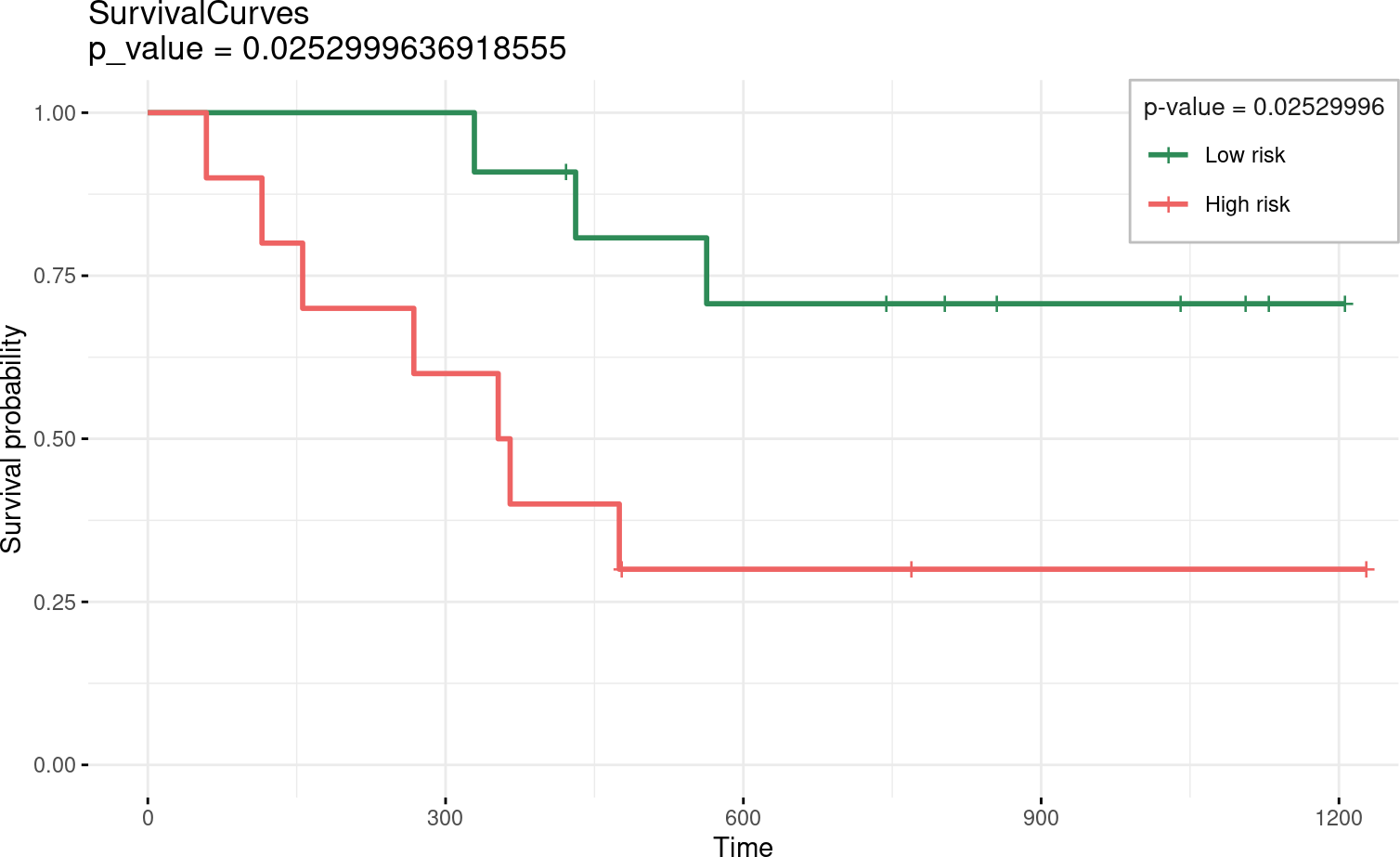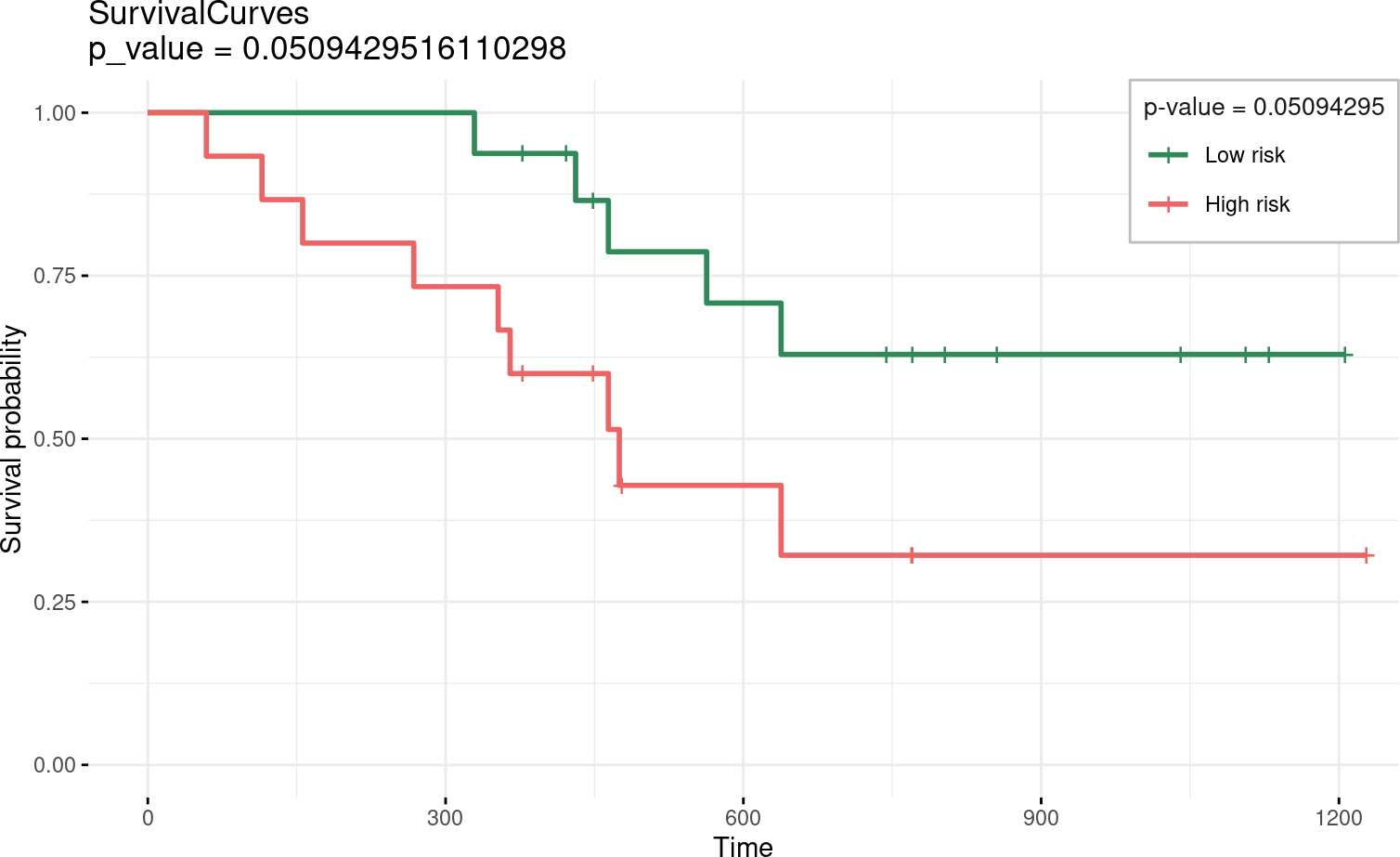# Contents

## 0.1 Instalation

if (!require("BiocManager"))
install.packages("BiocManager")
BiocManager::install("glmSparseNet")

# 1 Required Packages

library(futile.logger)
library(ggplot2)
library(glmSparseNet)

# Some general options for futile.logger the debugging package
.Last.value <- flog.layout(layout.format('[~l] ~m'))
.Last.value <- loose.rock::show.message(FALSE)
# Setting ggplot2 default theme as minimal
theme_set(ggplot2::theme_minimal())

## 1.1 Prepare data

data('ovarian', package = 'survival')
## Warning in data("ovarian", package = "survival"): data set 'ovarian' not found
xdata <- ovarian[,c('age', 'resid.ds')]
ydata <- data.frame(time = ovarian$futime, status = ovarian$fustat)

## 1.2 Separate using age as co-variate (group cutoff is median calculated relative risk)

res.age <- separate2GroupsCox(c(age = 1, 0), xdata, ydata)

### 1.2.1 Kaplan-Meier survival results

## Call: survfit(formula = survival::Surv(time, status) ~ group, data = prognostic.index.df)
##
##            n events median 0.95LCL 0.95UCL
## Low risk  13      4     NA     638      NA
## High risk 13      8    464     268      NA

### 1.2.2 Plot

A individual is attributed to low-risk group if its calculated relative risk (using Cox Proportional model) is below or equal the median risk.

The opposite for the high-risk groups, populated with individuals above the median relative-risk.## 1.3 Separate using age as co-variate (group cutoff is 40% - 60%)

res.age.40.60 <- separate2GroupsCox(c(age = 1, 0), xdata, ydata, probs = c(.4, .6))

### 1.3.1 Kaplan-Meier survival results

## Call: survfit(formula = survival::Surv(time, status) ~ group, data = prognostic.index.df)
##
##            n events median 0.95LCL 0.95UCL
## Low risk  11      3     NA     563      NA
## High risk 10      7    359     156      NA

### 1.3.2 Plot

A individual is attributed to low-risk group if its calculated relative risk (using Cox Proportional model) is below the median risk.

The opposite for the high-risk groups, populated with individuals above the median relative-risk.## 1.4 Separate using age as co-variate (group cutoff is 60% - 40%)

This is a special case where you want to use a cutoff that includes some sample on both high and low risks groups.

res.age.60.40 <- separate2GroupsCox(
chosen.btas = c(age = 1, 0),
xdata,
ydata,
probs = c(.6, .4),
stop.when.overlap = FALSE
)
## Warning in separate2GroupsCox(chosen.btas = c(age = 1, 0), xdata, ydata, : The cutoff values given to the function allow for some over samples in both groups, with:
##   high risk size (15) + low risk size (16) not equal to xdata/ydata rows (31 != 26)
##
## We are continuing with execution as parameter stop.when.overlap is FALSE.
##   note: This adds duplicate samples to ydata and xdata xdata

### 1.4.1 Kaplan-Meier survival results

## Kaplan-Meier results
## Call: survfit(formula = survival::Surv(time, status) ~ group, data = prognostic.index.df)
##
##            n events median 0.95LCL 0.95UCL
## Low risk  16      5     NA     638      NA
## High risk 15      9    475     353      NA

### 1.4.2 Plot

A individual is attributed to low-risk group if its calculated relative risk (using Cox Proportional model) is below the median risk.

The opposite for the high-risk groups, populated with individuals above the median relative-risk.